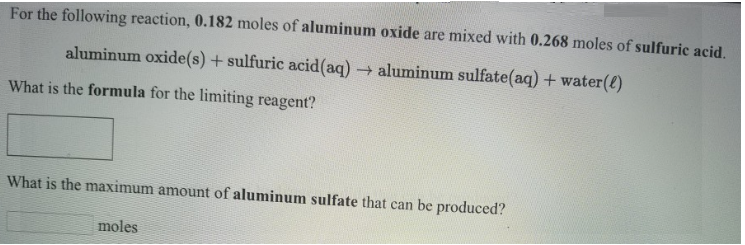# Problem: For the following reaction, 0.182 moles of aluminum oxide are mixed with 0.268 moles of sulfuric acid. aluminum oxide (s) + sulfuric acid (aq) → aluminum sulfate (aq) + water(l) What is the formula for the limiting reagent? What is the maximum amount of aluminum sulfate that can be produced?

###### FREE Expert Solution
90% (56 ratings)###### Problem Details

For the following reaction, 0.182 moles of aluminum oxide are mixed with 0.268 moles of sulfuric acid.

aluminum oxide (s) + sulfuric acid (aq) → aluminum sulfate (aq) + water(l)

What is the formula for the limiting reagent?

What is the maximum amount of aluminum sulfate that can be produced?